Latest SSC jobs   »   Reasoning Questions Quiz   »   Reasoning for SSC GD 2021: 17th...

# Reasoning for SSC GD 2021: 17th November

Directions (1-2): Choose the correct alternative that will replace the question mark.

Q1. 66, 36, 18, ?
(a) 3
(b) 7
(c) 9
(d) 8

Q2. 3, 12, 27, 48, 75, ?
(a) 108
(b) 162
(c) 156
(d) 142

Directions (3-5): Select the related letters/word/ number/figure from the given alternatives.

Q3. ACEG : IKMO : : QSUW : ?
(a) XBDF
(b) MOQS
(c) YACE
(d) BDFH

Q4. EHGI : LONP : : ? : ORQS
(a) GJIK
(b) GIHJ
(c) HKJL
(d) HJIK

Q5. 7584 : 5362 : : 4673 : ?
(a) 2367
(b) 2451
(c) 2531
(d) 2485

Directions (6): In the matrices a letter can be represented first by its row number and followed by its column number For example, A is represented by 12, 24; and R by 57, 76, etc. In each of the equations following matrices, identify one set of number pairs out of (A), (B), (C) and (D) which represents the given word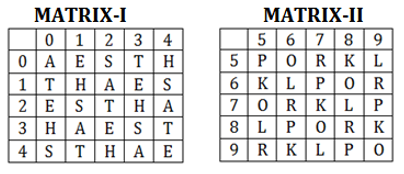Q6. EAST
(a) 32, 31, 02, 04
(b) 20, 43, 33, 11
(c) 13, 12, 14, 10
(d) 44, 32, 21, 03

Q7. Which one set of letters when sequentially placed at the gaps in the given letter series shall complete it?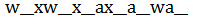(a) axawxa
(b) aawaxx
(c) aawwxx
(d) waawxx

Q8. A word is represented by only one set of numbers as given in any one of the alternatives. The sets of numbers given in the alternatives are represented by two classes of alphabets as shown in the given two matrices. The columns and rows of Matrix-I are numbered from 0 to 4 and that of Matrix-II are numbered from 5 to 9. A letter from these matrices can be represented first by its row and next by its column, for example, ‘P’ can be represented by 02, 10, etc., and ‘G’ can be represented by 68, 98, etc. Similarly, you have to identify the set for the word “TRAIL”.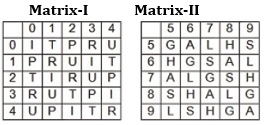(a) 01, 03, 75, 00, 68
(b) 14, 30, 68, 13, 58
(c) 20, 44, 99, 21, 96
(d) 43, 11, 56, 34, 88

Q9. In each of the following question below are given some statements followed by some conclusions. Taking the given statements to be true even if they seem to be at variance from commonly known facts, read all the conclusions and then decide which of the given conclusion logically follows the given statements.
Statements:
I. All cups are vegetable.
II. All vegetable are pens.
Conclusions:
I. Some pens are vegetable.
II. Some pens are cups.
(a) Only conclusion (I) follows.
(b) Only conclusion (II) follows.
(c) Both conclusion follow.
(d) Neither conclusion (I) nor conclusion (II) follows.

Q10. The ratio of present ages of P and Q is 5 : 8. Three years later their ages will be in ratio 8: 11. What is the present age (in years) of Q?
(a) 5
(b) 11
(c) 14
(d) 8

Solutions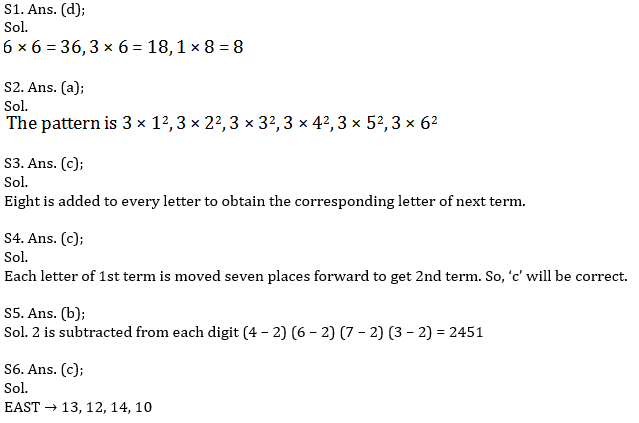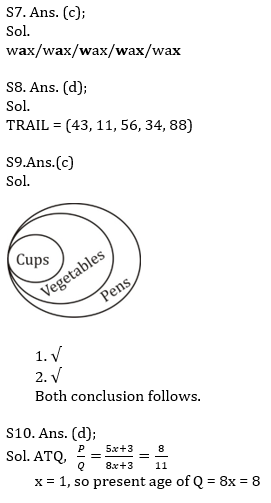#### Congratulations!General Awareness & Science Capsule PDF